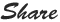## PHP Sort : How to write PHP sort for array

Simple sort keyword to sort array in sorted order

Syntax :

<!DOCTYPE html>
<html>
<title>  Simple PHP Array Sort for Alphabet </title>
<body>

<?php
\$alphabets= array("a""b""c");
sort(\$alphabets);

\$alph_length= count(\$alphabets);
for(\$i = 0; \$i < \$alph_length; \$i++) {
echo \$alphabets[\$i]
."<br>";

}
?>

</body>
</html>

Output:
a
b
c

<!DOCTYPE html>
<html>

<title>  Simple PHP Array Sort for Number </title>
<body>

<?php
\$numbers= array(132);
sort(\$numbers);

\$num_length= count(\$numbers);
for(\$i = 0; \$i < \$num_length; \$i++) {
echo \$numbers[\$i]."<br>";

}
?>

</body>
</html>
Output:
1
2
3## Writing PHP Array Function

PHP array : array can store multiple values in it, below are different scenarios are given  to understand how to write array,

Scenario1

<html>
<body>
<title> Writing PHP Array Function </title>
<?php
\$fruit = array("Mango","Apple","Banana","Kiwi");
var_dump(\$fruit);
?>
</body>
</html>

Output :
array(4) { => string(6) "Mango" => string(4) "Apple" => string(5) "Banana" => string(3) "Kiwi" }

scenario2
<html>
<body>
<title> Writing PHP Array Function </title>
<?php
\$fruit = array("Mango","Apple","Banana","Kiwi");
print_r(\$fruit);
?>
</body>
</html>

Output :
array(4) { => string(6) "Mango" => string(4) "Apple" => string(5) "Banana" => string(3) "Kiwi" }

scenario3
<html>
<body>
<?php
/* First Scenario to create array. */
\$numbers = array( 55, 66, 77, 88, 99);
foreach( \$numbers as \$value ) {
echo "Value is \$value <br />";
}
?>
</body>
</html>
Output :
Value is 55
Value is 66
Value is 77
Value is 88
Value is 99

Scenario4
<html>
<body>
<?php
/* Second Scenario to create array and assign values to it. */
\$fruit = "mango one";
\$fruit = "banana two";
\$fruit = "apple three";
\$fruit = "kiwi four";
\$fruit = "greps five";

foreach( \$numbers as \$value ) {
echo "Fruit value is \$value <br />";
}
?>
</body>
</html>
Output :

Fruit value is mango one
Fruit value is banana two
Fruit value is apple three
Fruit value is kiwi four
Fruit value is greps five## PHP Float Data type : How to write float in PHP?

PHP Float Data Type :

Float is number with decimals, any number with decimal point can be considered as float datatype.

Below example have \$f as float. and the function PHP var_dump() returns the value value of float.

Syntax:

<!DOCTYPE html>
<html>
<body>

<?php
\$f = 85.969;
var_dump(\$f);
?>

</body>
</html>

Output :

float(85.969)

--------------------## PHP Integer

Integer has few rules, before writhing integer we need to consider those rules first.

Integer should be least one digit, and it should not have decimal point in place. only positive or negative integer can be considered.

An integer has limit from -2,147,483,648 and 2,147,483,647.

Syntax :

<!DOCTYPE html>
<html>
<body>

<?php
\$x = 1234;
var_dump(\$x);
?>

</body>
</html>

Output :

int(1234)## PHP Data Types

There are 9 types of PHP datatypes available those can store the data into it.
• Integer
• Float
• String
• Boolean
• Resource
• Array
• Object
• NULL
Notes: Float data type can be used as "double" data type..

## PHP String......

<!DOCTYPE html>
<html>
<body>

<?php
\$i = "All New";
\$j = 'PHP 7 is Here';

echo \$i;
echo "<br>"
echo \$j;
?>

</body>
</html>

Output :

All New
PHP 7 is Here## The PHP foreach Loop

The foreach loop condition is different that for look,  to execute foreach look , the array condition is must , each array parameters is works as "key" point for for each loop ,below code will explain  much better.

Syntax :

foreach (\$yourArray as \$keyValue) {
\$KeyValue handle code to be executed here;
}

#### Code :

<!DOCTYPE html>
<html>
<body>

<?php
\$Fruits= array("mango""apple""banana""kiwi");

foreach (\$Fruits as \$keyValue) {
echo "\$KeyValue <br>";
}
?>

</body>
</html>

Output :

mango
apple
banana
kiwi

Scenario : If condition under foreach loop.

<!DOCTYPE html>
<html>
<body>

<?php
\$Fruits= array("mango""apple""banana""kiwi");

foreach (\$Fruits as \$keyValue) {

if(\$keyValue=="banana")
{
echo "I like banana"
}

}
?>

</body>
</html>

Output :
"I like banana"## PHP for loop

For loop can be written in three parameters,  first "initial counter", second "condition counter"  third "increment counter"

Syntax :

for (initial counter; condition counter; increment counter) {
white for loop actual code here.....
}

Code :

<!DOCTYPE html>
<html>
<body>

<?php
for (\$i = 0; \$i <= 10; \$i++) {
echo "Counter : \$i <br>";
}
?>

</body>
</html>

Output :

Counter : 1
Counter : 2
Counter : 3
Counter : 4
Counter : 5
Counter : 6
Counter : 7
Counter : 8
Counter : 9
Counter : 10